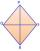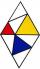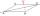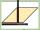# Diagonals in diamons/rhombus

Rhombus ABCD has side length AB = 4 cm and a length of one diagonal of 6.4 cm. Calculate the length of the other diagonal.

Result

u2 =  4.8 cm

#### Solution:Leave us a comment of example and its solution (i.e. if it is still somewhat unclear...):

Showing 0 comments:Be the first to comment!#### To solve this verbal math problem are needed these knowledge from mathematics:

Pythagorean theorem is the base for the right triangle calculator.

## Next similar examples:

1. DiamondCalculate the length of the two diagonals of the diamond if: a = 13 cm v = 12 cm
2. RhombusCalculate the length of the diagonal AC of the rhombus ABCD, if its perimeter is 84 dm and the other diagonal BD has length 20 dm.
3. ParkIn the park is marked diamond shaped line connecting locations A, D, S, C, B, A. Calculate its length if |AB| = 108 m, |AC| = 172.8 m.
4. ConstructConstruct a rhombus ABCD, if the size of the diagonal AC is 6 cm and diagonal BD 8 cm long.
5. Rhombus OWESOWES is a rhombus given that OW 6cm and one diagonal measures 8cm. Find its area?
6. SatinSanusha buys a piece of satin 2.4 m wide. The diagonal length of the fabric is 4m. What is the length of the piece of satin?
7. Tv screenThe size of a tv screen is given by the length of its diagonal. If the dimension of a tv screen is 16 inches by 14 inches, what is the size of the tv screen?
8. UmbrellaCan umbrella 75 cm long fit into a box of fruit? The box has dimensions of 390 mm and 510 mm.
9. CablewayCableway has a length of 1800 m. The horizontal distance between the upper and lower cable car station is 1600 m. Calculate how much meters altitude is higher upper station than the base station.
10. Chord circleThe circle to the (S, r = 8 cm) are different points A, B connected segment /AB/ = 12 cm. AB mark the middle of S'. Calculate |SS'|. Make the sketch.
11. Cone 15The radius of the base of a right circular cone is 14 inches and it's height 18 inches. What is the slant height?
12. Broken treeThe tree was 35 meters high. The tree broke at a height of 10 m above the ground. Top but does not fall off it refuted on the ground. How far from the base of the tree lay its peak?
13. Double ladderThe double ladder is 8.5m long. It is built so that its lower ends are 3.5 meters apart. How high does the upper end of the ladder reach?
14. WindbreakA tree at a height of 3 meters broke in the windbreak. Its peak fell 4.5 m from the tree. How tall was the tree?
15. Double ladderThe double ladder shoulders should be 3 meters long. What height will the upper top of the ladder reach if the lower ends are 1.8 meters apart?
16. Common chordTwo circles with radius 17 cm and 20 cm are intersect at two points. Its common chord is long 27 cm. What is the distance of the centers of these circles?
17. HeightIs right that in any right triangle height is less or equal half of the hypotenuse?